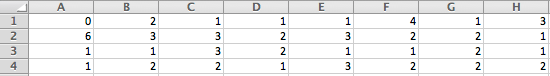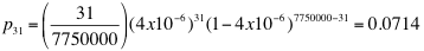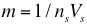Hemocytometer

Many home brewers use a hemocytometer to count the density of yeast cells in pitched wort in order to see if they are complying with the rule of thumb that there should be 1 million cells per mL per °P. On this page we'll look at two aspects of this. The first is how to carry out a count and the second the implication of the number of cells counted.

We start with a photomicrograph of the grid of a hemocytometer. These are ruled in various ways depending on manufacturer but we can make the general remarks that there is typically a central counting area that is 1 mm x 1 mm ruled into 25 squares each 0.2 mm x 0.2 mm marked by triple line boundaries (see photo). These are subdivided into 16 small squares of 0.05 x 0.05 mm. When a cover slip is placed on the slide it is held up off the ruled surface by typically 0.100 mm (this is usually marked on the slide) so that the volume of liquid trapped between the cover slip within the boundaries of one of the triple ruled (large) squares is 0.0002 x 0.0002 x 0.0001 = 4 x 10-12 mwhich is 4 x 10-6 mL (4 nL).  The large square is defined by the triple ruled lines or rather by the center of the three lines in the triple ruling. If you look closely you will see that the small squares bounded on one or two sides by triple ruling center lines are the same size as the other small squares (delimited by single ruled lines). Thus when counting squares with a triple line the center line should be used.

Each small square contains a volume 1/16 that of a large square which in the case of this hemocytometer is 2.5 x 10-7 mL (.25 nL).As the triple lines are (center to center) 0.2 mm apart on the slide you can determine the magnification of the picture you are looking at by measuring the distance between parallel triple lines on the monitor or paper. If you measure 50 mm then you have an effective magnification of 250X. You can get an idea as to the size of a yeast cell from this. They seem, at 250X, to measure about 1.5 mm in diameter and this the actual cell is 1/250th of this or 0.006 mm (6 microns) in diameter.

In counting cells we must have a rule that assigns each cell to one and only one square. The usual rule is that if a cell touches a boundary line at all i.e. even if it is only tangent to it, you include it in the box bound by that line if the line is an upper line or left hand line and exclude it if it is a lower or right hand line. To illustrate this designate the small squares in the row just below the uppermost horizontal triple in the photo as A1, B1, …H1; the squares in the row below that as A2…H2 and so on. Small square A1, by the given counting rule, contains no cells because the only one in it touches the lower boundary line. That cell will be counted when we count square A2 because it touches the upper line in that square. Square A2 contains 2 cells (the small dot is not a yeast cell), square A3 1, square A4 1, square A5 1, square A6 from 2 to 4. The small cell touching the center line of the triplet at the top is smaller than the other cells. Is it a yeast cell? Is it a yeast cell of the same strain i.e. a small daughter cell or some other strain? There are times when you will have to make judgements like this. Now look at he cell touching the right hand wall of the square. Is it touching the wall or not? Probably not - the resolution of the microscope isn't sufficient to tell us. In any case none of it crosses the line into square G1's area and when we count square G1 we probably wouldn't think of it as touching the line. In this case we can mentally move the cell further into F1 but we must remember that we did this. On the other hand if we decide that this cell is touching the line we must remember that we made that decision when we count G1. F1 plainly contains 1 cell and G1 contains 3, all touching the upper triple line center. Now look at square E4 in particlar at the cell at the lower left corner. We include it here because it touches the left boundary but not the bottom boundary. But suppose it did. Suppose it to be a perfect circle that surrounds the intersection of this lower left corner. Parts of it appear in four cells. Which one do we assing it to? We would clearly exclude it from D4 because it touches both a right and bottom boundary. But how about the square below A4? It touches an upper and a right boundary there. The answer is that contact with a disqualifying boundary overrides a qualifying one and we would reject it. In square E4 the same situation applies - it is in contact with a diqualifying boundary (the bottom) and is therefore rejected. In the square below E4, however, it contacts only qualifying boundaries and is assigned, therefore, to that square.

In the following table we list our cell counts for the picture above. See if you agree with them.The total count is 62 over 32 cells of  2.5 x 10-7 mL (.25 nL) each for a total volume of 8 x 10-6 mL so that the estimated density is 62/(8 x 10-6) = 7.75 x 106 cells/mL.

We want to illustrate the questionable cell location rule a little more clearly. We wanted to get the table with the counts and the photograph of the actual hemocytometer close enough together on the screen that you would be able to see them both and so held off on the discussion which follows

In the image here you see large square boundaries and three 'cells': a black one, a red one and a blue one. Text of like color located in a square describes why the cell should or should not be assigned to that square. Remember that the goal is to assign each cell to one square and one square only. Restated more concisely the rule is

A cell which touches a boundary line (or lines) is assigned to the square bounded by that line (those lines) if it (they) bound the square from the left or above unless it also touches a line that bounds from below or the right. A cell which touches a line or lines bounding a square from the right or below is not assigned to that square.

This rule is completely illustrated by examining the four squares surrounding the black 'cell'.It's quite clear that the red cell should be assigned to the square to the right of the line it touches and as this one is clearly half in this square and half in the one to the left of the boundary you should be comfortable, in the spirit of the rule's intent, to assing it to the right square. But how about the blue cell? It is clearly in the left square but it does touch a boundary to the right. Or does it? Remember that there was a similar cell in the photomicrograph. As we noted there you can either put this cell in the left square or the right one just as long as you don't put it in both. And you can do this by making a decision as to whether it actually touches the boudary line or not. If you decide it does then it goes in the right square by strict observation of the rule. If you decide it doesn't it goes in the left square. The danger in a situation like this is that you may decide it is  touching the line when you count the left square (so that you do not count it) but then, when counting the right square, not look at it closely because at first glance it appears to be completely in the left square and so not count it here either. The conclusion is that you must force yourself to look at the boundary lines themselves - not just focus on the interior of the squares they describe.

Now let us interpret the counts from the photomicrograph. For this hemocytometer the depth is 0.1 mm. Thus each large square overlies a volume of  0.0002 x 0.0002 x 0.0001 = 4 x 10-12 m3 which is 4 x 10-6 mL  (a liter is 10cm x 10 cm x 10 cm and a cubic meter thus contains 1000 of those at 1000 mL apiece). Each of the large squares in the photo contains, by amazing coincidence, 31 cells. Thus the cell density is 31/(4 x 10-6) = 7,750,000 cells per mL (note that this is old beer that has been disturbed - not freshly pitched wort). Or is it. Let us suppose for the moment that it is and look at the probabilty that we get 31 cells in a volume corresponding to 1 large square (4 x 10-6 mL). There are 250,000 such volumes in a mL so that the probability that an individual cell winds up in the one we are counting is 4 x 10-6 and the probability that we get 31 in that cell isor 7% which implies that there is a 93% chance that we get some other number. This is why we are surprised that we got 31 twice. The probability of that happening, if the density is really 7750000 cells /mL, is 0.005 or 1 in 200. The object with the 31 and the 7750000 is the binomial coefficient and the probabilities are binomially distributed. For such large numbers, however, we can approximate the binomial distrubution by the Gaussian with mean given byand standard deviation bywith the approximation valid because p is a small number. Notice that the standard deviation is the square root of the mean. Thus for 7750000 cells per mL and a 4x10-6  mL volume leading to p of 4x10-6 we would expect a mean count of 31 and a standard deviation of 5.6. The counts we would see would fall between 31 - 6 and 31 + 6 approximately 68% of the time causing us to conclude that the cell density is somewhere between 6,250,000 and 9,250000. This amounts to ±18% error.

Suppose now that we count 2 squares instead of 1. The probability, p, that a given cell falls in the volume of 2 squares is double the probability that it falls in the volume of 1 and the mean for the count in two square is thus 62 (again assuming that the true density is 7750000 cells/mL). But the standard deviation does not double. It goes up by the square root of 2. Thus the error as a percentage decreases by a factor of sqrt(2) for each doubling of the number of squares read.

Let's generalize this. Suppose you have a hemocytometer whose large squares contain volume Vs (in mL) and that you propose to count ns large squares. Suppose also that the cell density is N cells per mL. In 1 mL there are'bins' of volume  nsVs   so the probability, p, that a cell will fall into any particular one isThe mean and standard deviation of the total number of cells we expect to count areand

and the ratio of standard deviation to mean (the fractional standard error) isThe ratio of the standard error in an estimate to its mean is is often called the 'coefficient of variation' and that's why we used Cv to represent it.

Note that NVs is the expected number of cells in each large square.

Lets suppose that we have brewed a 12°P beer and hope to have pitched it to the recommended 12 million cells per mL. What might we expect to see with a hemocytometer like the one in the photograph? With its large square volume of 4x10-6 mL we would expect (12 x 106)(4x10-6)= 48 cells per large square (or 3 per small square).

*under editing from here on *

For a single square counted we would expect the coefficient of variation to be 1/sqrt(48) = 0.149 i.e 14.9%. That's pretty good. But who wants to try to count 75 cells per small square through a microscope? The job will be a lot easier if we dilute say 4:1 so that the average number of cells in a small square is only 15 and in a large one 240. But 1/sqrt(240) = 6.45% which is sqrt(5) times 2.9%. Clearly the way to get back to 2.9% is to count 5 large squares and equally clearly the bottom line is that the accuracy depends on the number of cells you count however you count them.

The Coefficient of Variation

The coefficient of variation gives is the standard devation normalized by the mean. Multiplied by 100 it expresses the standard deviation as a percent of the mean. It is important to understand that what we have done is count the number of cells and convert that into a number of cells per mL estimate based on the volume of a square. To calculate the Cv we assume that the cell density based on the count is the actual cell count and then calculate the standard deviation based on that assumption using the binomial distribution of the number of cells that would be observed conditioned on the density being the hypothesized density. We can turn this around (using Bayes Theorem) to compute the probaility of the actual density based on the number of cells we counted. The density value that gives the highest probability is the most likely actual density. It is the number of cells counted divided by the volume counted. This is called a maximum liklihood estimator of the number of cells per mL. The probability distribution of actual cell density conditioned on the number we observed is very nearly a Gaussian distribution. Thus by referring the Cv to a Gaussian distribution we can calculate the probabiluty that the actual cell count lies betwee desired limits.

As an example let us suppose we counted 1000 cells in 5 large squares each of which has volume 10-4 mL. The average density is then 1000/5x 10-4  2,000,000 cells/ml. Thw  is Cv = 1/sqrt(1000) = 3.16%. Referring to values for the Gaussian  standard error distibution we can say that the actual cell density was within ± 3.16% ( 1,936,800 to 2,072,000) of 2,000,000 cells per mL with probability 68%, that it is within ± 6.32% of 2,000,000 with probability 95.4% and within 9.48% of 2,000,000 with probability 99.6% .

If tighter uncertainly bounds are required (e.g. we wish 2.9% error with 95% cerainty) we would need to halve the standard deviation which means quadrupling the number of large squares counted.